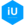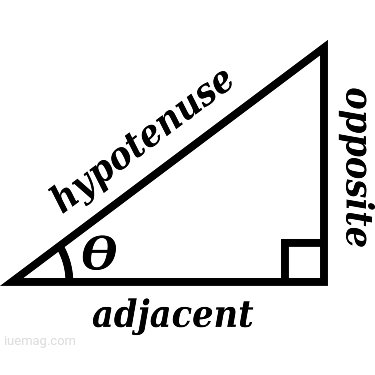Inspiration Corner
A collection of write-ups from experiences of life that inspire, empower, influence & trigger positive values, habits & mindsets.

# The Main Formulas, Properties, And Uses Of TrigonometryIntroduction: In Mathematics triangle is a very common figure that you learn in geometry. Students at a certain age need to learn the properties of these figures. Even when you draw you have to remember these features carefully. But when you combine the properties of the angles with sides, you will get trigonometry. With this, you can find the length of the sides if you gather the value of one angle. Besides these, there are other factors such as the hypotenuse. It is the side with the maximum size and is located just opposite 90 degrees. As a result, you get a string of trigonometry formulas that define all these features.

### Basic elements:

It is a process that students can use to find angles whose values they don't know. You need to learn the formula or a few symbols that correspond to a fixed ratio. For example, when you consider the sine of an angle, it means the ratio of the perpendicular line with the hypotenuse. If you keep increasing the shape of the triangle, this ratio will remain the same. Similarly, there are other formulas such as cosine, secant, etc. But you can apply these properties only when one angle of the triangle is equal to 90 degrees.

### Some basic features:

Students have to learn the formula for each symbol in trigonometry. But there are three main functions that they need to use constantly. They include the sin, cos, and tan values of the angle present in the paper. If you know these three the remaining you can derive easily. All you need to do is find out the inverse of them and get three remaining formulas. The value of these ratios can lie in any range. However, there are certain fixed angles whose trigonometric values must be learned. These include the angle of 45 degrees, 30 degrees, etc. You don't need to put in the size of the sides each time you do a sum. If you find one of these fixed angles you can directly write the values.

###Properties that you have to know:

Some common properties build the basics of trigonometry. Up to one stage you have to calculate the angles and ratio. But after that, you have to solve several equations based on these properties. One of them is the fact that the sin^2 and cos^2 of any angle will always be one. It is a universal fact and you cannot change this by any means. Even when you derive other formulas you can apply these properties to get the result quickly.

Along with properties, students have to catch up with trigonometric identities. For example, you can get the value of sin(A + B). As a result, you can break the angles into two parts and apply the formula to find these values. First, you have to multiply sin A with Cos B and then cos A with Sin B. Add the two results and you will get the final answer directly. Similarly, there is a long-range formula for tan ( A + B) as well. Students usually forget that the sign can change the entire structure of the formulae.

The major applications of this chapter:
The main region where people use trigonometry is heights. You can easily find out the height of a pole if you consider the angle of the shadows. Even in mountain regions, people use this process. You will learn a chapter where there are only sums related to this chapter. Most of them will revolve around the distance between two buildings. You will be shocked to know how important trigonometry is around you. For obtaining detailed knowledge you can learn trigonometric identities directly from Cuemath.